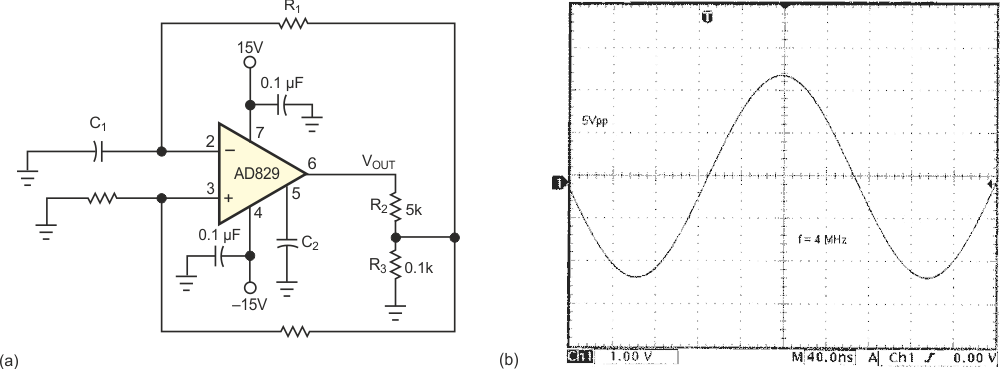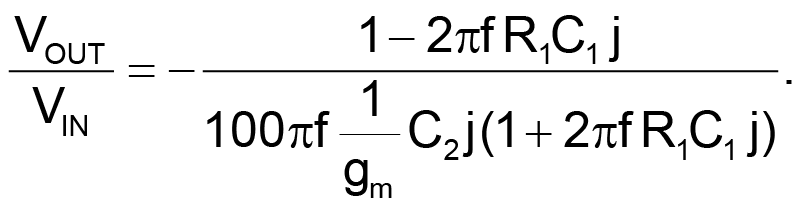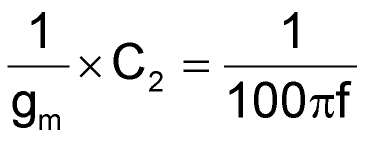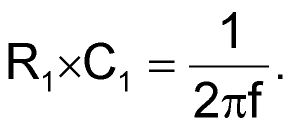# Amplitude-stable oscillator has low distortion, low cost

Moshe Gerstanhaber

EDN

The multivibrator is a common circuit that consists of an amplifier with both positive and negative feedback (Figure 1a). When the output is positive, the positive input terminal equals ½V+, and the voltage at the negative input terminal changes toward V+. When this voltage exceeds ½V+, the output voltage rapidly changes to V–. The positive input terminal becomes ½V–, and the negative input terminal changes toward V–. When the voltage at the negative input terminal is less than ½V–, the process repeats (Figure 1b).Figure 1. The common multivibrator has positive and negative feedback (a). When VOUT is positive, VIN– changes toward V+. When VIN– exceeds V+/2, VOUT changes to V– (b).

For the multivibrator to work, the bandwidth of the amplifier must be 10 times higher than the time constant of the passive network, and consideration of the high slew rate helps define the amplifier. The output is a square wave.

The circuit in Figure 2a is a sinusoidal oscillator. External compensation at Pin 5 forces the unity-gain bandwidth of the amplifier to be the same as the passive-network bandwidth.Figure 2. This stable oscillator (a) has low distortion (b).

Loop-gain analysis results in the following transfer function:To meet the conditions necessary to sustain oscillation – loop gain equal to unity and phase equal to zero – chooseandThe inverse transconductance, 1/gm, of the input stage, re, is equal to 52 Ω. The design assures amplitude stability because re always increases with an amplitude increase, which reduces the loop gain. The ratio of the R2/R3 divider network sets the amplitude.

Figure 2b is a performance photo of the oscillator running at 4 MHz and 5 V p-p. For better frequency stability, you can replace C2 with a crystal of the desired frequency and low shunt capacitance.

## Materials on the topic

EDN